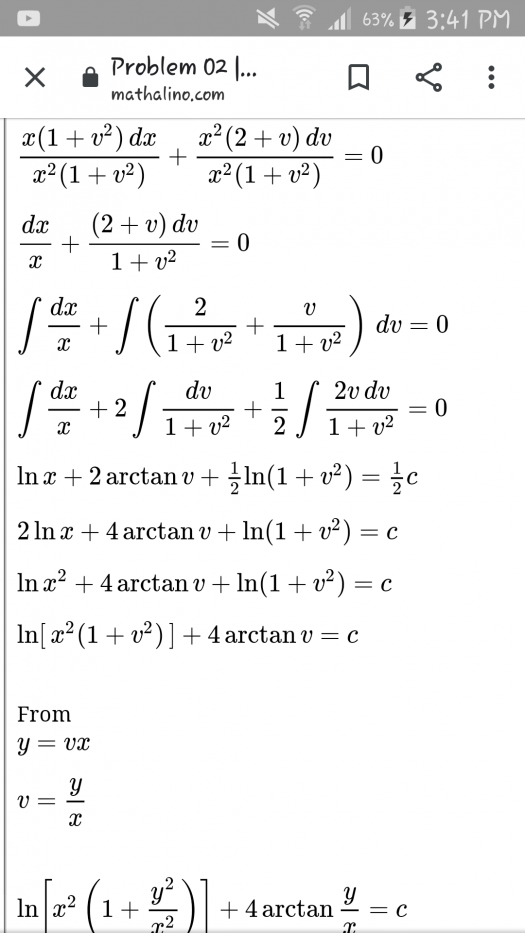# diff eqn

2 posts / 0 new
Kennett Rubiadiff eqnPAANO PO NAGKAROON NG 1/2 ANG CONSTANT AFTER NG INTEGRATION ????

Jhun Vert$\frac{1}{2}c$, $\frac{1}{4}c$, $\ln c$, $\tan c$, $\sqrt{c}$, $e^c$, $c^2$, $\pi c$; it doesn't matter, it is still a constant term. Do as you wish, but I suggest you write your constant term in a way that it will help you write the equation in a more elegant way.

• Mathematics inside the configured delimiters is rendered by MathJax. The default math delimiters are $$...$$ and $...$ for displayed mathematics, and $...$ and $...$ for in-line mathematics.